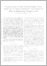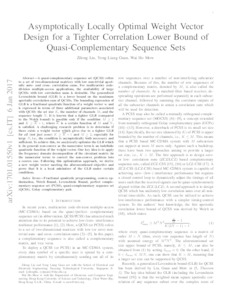# Asymptotically Locally Optimal Weight Vector Design for a Tighter Correlation Lower Bound of Quasi-Complementary Sequence Sets

Liu, Zilong and Guan, Yong Liang and Mow, Wai Ho (2017) 'Asymptotically Locally Optimal Weight Vector Design for a Tighter Correlation Lower Bound of Quasi-Complementary Sequence Sets.' IEEE Transactions on Signal Processing, 65 (12). 3107 - 3119. ISSN 1053-587XPreview
Text
1701.01550.pdf - Accepted Version

Download (1MB) | Preview

## Abstract

A quasi-complementary sequence set (QCSS) refers to a set of two-dimensional matrices with low nontrivial aperiodic auto- and cross-correlation sums. For multicarrier code-division multiple-access applications, the availability of large QCSSs with low correlation sums is desirable. The generalized Levenshtein bound (GLB) is a lower bound on the maximum aperiodic correlation sum of QCSSs. The bounding expression of GLB is a fractional quadratic function of a weight vector w and is expressed in terms of three additional parameters associated with QCSS: the set size K, the number of channels M, and the sequence length N. It is known that a tighter GLB (compared to the Welch bound) is possible only if the condition M ≥ 2 and K ≥ K̅ + 1, where K̅ is a certain function of M and N, is satisfied. A challenging research problem is to determine if there exists a weight vector that gives rise to a tighter GLB for all (not just some) K ≥ K̅ + 1 and M ≥ 2, especially for large N, i.e., the condition is asymptotically both necessary and sufficient. To achieve this, we analytically optimize the GLB which is (in general) nonconvex as the numerator term is an indefinite quadratic function of the weight vector. Our key idea is to apply the frequency domain decomposition of the circulant matrix (in the numerator term) to convert the nonconvex problem into a convex one. Following this optimization approach, we derive a new weight vector meeting the aforementioned objective and prove that it is a local minimizer of the GLB under certain conditions.

Item Type: Article Faculty of Science and Health > Computer Science and Electronic Engineering, School of Elements 05 Oct 2020 16:23 05 Oct 2020 16:23 http://repository.essex.ac.uk/id/eprint/26512

### Actions (login required)View Item